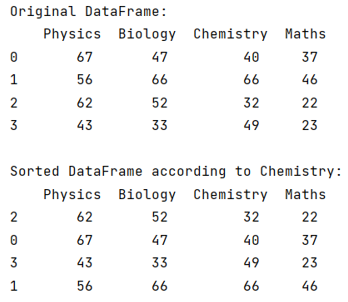# DataFrame object has no attribute sort

Solution for "DataFrame object has no attribute sort".
By Pranit Sharma Last updated : September 19, 2023

Pandas is a special tool that allows us to perform complex manipulations of data effectively and efficiently. Inside pandas, we mostly deal with a dataset in the form of DataFrame. DataFrames are 2-dimensional data structures in pandas. DataFrames consist of rows, columns, and data.

Sorting is a process of arranging the data according to our ease. Sorting is a process in which we can arrange the data either in ascending order or in descending order.

## Solution for DataFrame object has no attribute sort

To sort values in pandas DataFrame, we will first create a Dataframe and then we will sort the values according to some specific column. In pandas, we can sort the column values as well as row values, we use DataFrame.sort_values() method by specifying the column name as parameter.

Note

To work with pandas, we need to import pandas package first, below is the syntax:

```import pandas as pd
```

Let us understand with the help of an example,

## Python solution for 'DataFrame object has no attribute sort'

```# Importing pandas package
import pandas as pd

# Creating a dictionary
d = {
'Physics':[67,56,62,43],
'Biology':[47,66,52,33],
'Chemistry':[40,66,32,49],
'Maths':[37,46,22,23]
}

# Creating a DataFrame
df = pd.DataFrame(d)

# Display original DataFrame
print("Original DataFrame:\n",df,"\n")

# Sorting values according to Chemistry column
result = df.sort_values(by='Chemistry')

# Display result
print("Sorted DataFrame according to Chemistry:\n",result)
```

### Output

The output of the above program is: